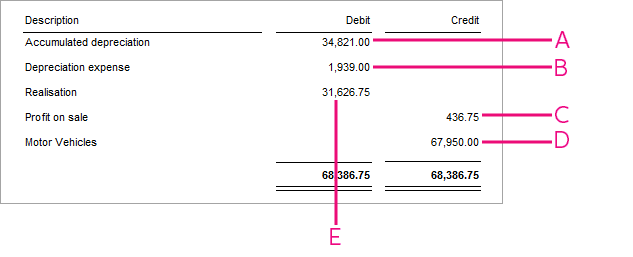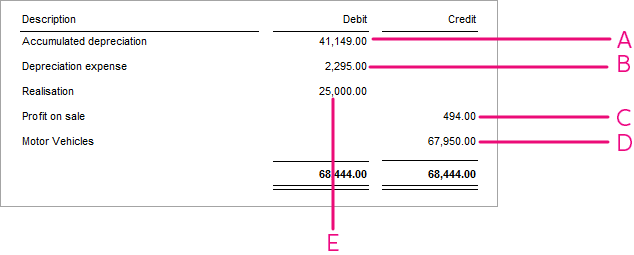##### Page tree

Assets performs several calculations to make up the journal entry for the sale. The calculation of profit or loss on the sale of an asset may differ.

For example, consider the following scenario:

BMW 3 Series Sedan
Taxation details
Accounting details
Current financial year20182018
Date asset began depreciating27/03/201427/03/2014
Original cost\$ 67,950\$ 67,950
Motor vehicle cost limit at the year of purchase (AU only)\$ 57,466N/A
Opening written down value (OWDV)

\$ 22,645 **

\$ 26 801
Depreciation rate / method25% diminishing value25% diminishing value
Date of sale02/11/2017 (125 days held this FY)02/11/2017 (125 days held this FY)
Consideration\$ 25,000\$ 25,000

** 2016 is a leap year for taxation purposes (366 days held / 365 days in a year). The ATO cost limit for the 2014 financial year is \$57,466.

Taxation journal explainedDescriptionFormulaExample
A

Write back accumulated depreciation

Original cost – opening written down value (OWDV)

Motor vehicle (MV) cost limit – OWDV (for vehicles subject to cost limit)

57,466 – 22,645

= 34,821

B

Apply depreciation for the current year, up to the date of sale

(NZ) In the year a Taxation Asset is sold, no depreciation for that year is applied.

OWDV * rate * days held / days in the year

22,645 * 0.25 * 125 / 365

= 1938.78

C

i). Calculate closing adjusted value (CAV)

OWDV – Accumulated depreciation for days held this financial year

22,645 - 1938.78

= 20,706.22

ii). Calculate adjusted termination value (if applicable)

[(MV cost limit + second element costs) / total cost of car] * termination value

(see ITAA97 s 40-325 and ITAA97 s 40-320)

[(57466 + 0) / 67950] * 25000

= 21,143

iii). Calculate the assessable profit or loss on sale for tax purposes

21,143 - 20706.47

= 436.75 **

D

Write back original cost of the asset.

EBalance the journal by offsetting the net to the Realisation account.

** subject to rounding

Accounting journal explainedDescriptionFormulaExample
A

Write back accumulated depreciation.

Original cost – opening written down value (OWDV)

67950 – 26801 = 41,149

B

Apply depreciation for the current year, up to the date of sale

OWDV * rate * days held / days in the year

26801 * 0.25 * 125 / 365 = 2294.62

C

Calculate closing adjusted value (CAV), then calculate the profit or loss on the sale.

OWDV – Accumulated depreciation for days held this financial year